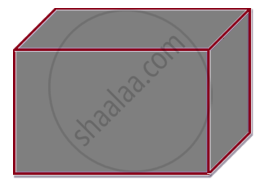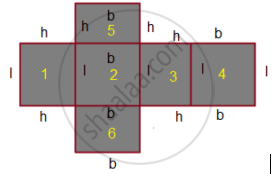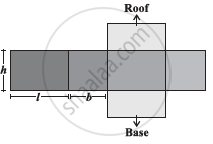Share

# Surface Area and Volume of Cube, Cuboid and Cylinder - Concept of Cuboid

#### notes

⦁ Surface area means all the area that you can see in two dimensions - this means the length and width.
⦁ Total surface area in that case means adding up the areas of all the surfaces.

For a cube, that means adding up the surface area of all six sides. For a sphere like a baseball, you want to know how much area the leather casing measured.

(i) Surface area of Cuboid :
A cuboid is a three-dimensional representation of a rectangle.Cuboid is an assemblage of rectangular pieces as length, breadth, and height.A cuboid when opened gives the following view:The figure above shows that a cuboid is made of six rectangles (where, l,b, and h are the three edges of a cuboid). The area of the six rectangles in consecutive order from 1 to 6 are:

1) area = (l × h)
2) area = (l × b)
3) area = (l × h)
4) area = (l × b)
5) area = (b × h)
6) area = (b × h)

When we sum up all the areas we get the surface area of the cuboid = 2(l x b) +2(b x h) + 2(l x h) =2(lb+bh+hl). The surface area of a cuboid hence is:

surface area of a cuboid = 2 (lb + bh + hl)

Lateral Surface Area of Cuboid :The side walls (the faces excluding the top and bottom) make the lateral surface area of thecuboid.

For example, the total area of all the four walls of the cuboidal room in which you are sitting is the lateral surface area of this room .

Hence, the lateral surface area of a cuboid is given by
= 2(h × l + b × h) or 2h (l+ b).

S